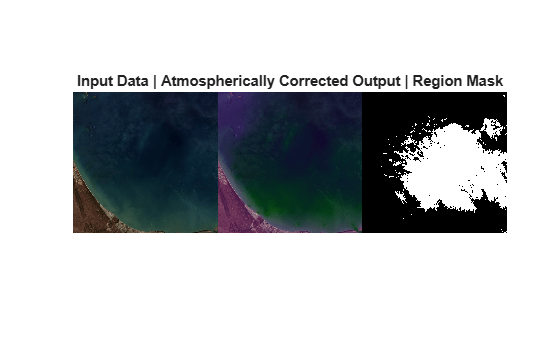# rrs

Compute remote sensing reflectance

Since R2020b

## Syntax

``newhcube = rrs(hcube)``
``[newhcube,mask] = rrs(hcube)``

## Description

````newhcube = rrs(hcube)` computes remote sensing reflectance (RRS) values. The remote sensing reflectance values are the atmospherically corrected values. The function first computes the water leaving radiance and then, estimates RRS as the ratio of water leaving radiance to solar irradiance at the top of the atmosphere (TOA).The pixels values of the data cube must be TOA radiance values. If the values are digital numbers, use the `dn2radiance` function to compute the TOA radiance values. This method gives best results for multispectral data.```

example

````[newhcube,mask] = rrs(hcube)` also returns a region mask indicating the clear water regions in the input satellite data. NoteThis function requires the Image Processing Toolbox™ Hyperspectral Imaging Library. You can install the Image Processing Toolbox Hyperspectral Imaging Library from Add-On Explorer. For more information about installing add-ons, see Get and Manage Add-Ons.The Image Processing Toolbox Hyperspectral Imaging Library requires desktop MATLAB®, as MATLAB Online™ or MATLAB Mobile™ do not support the library. ```

## Examples

collapse all

Read multispectral data into the workspace.

`hcube = hypercube('LC08_L1TP_097070_20201101_20201101_01_cropped.dat');`

Convert the pixel values from digital numbers to top of atmosphere (TOA) radiances.

`hcube = dn2radiance(hcube);`

Estimate remotely sensed water reflectance using the clear-water pixels approach.

`[newhcube,mask] = rrs(hcube);`

Estimate RGB images of the input and the atmospherically corrected output data.

```imgIn = colorize(hcube,'Method','rgb'); imgOut = colorize(newhcube,'Method','rgb');```

Display the RGB images of the input and the atmospherically corrected output data. Also, display the clear-water pixel region mask used for computing the correction parameters.

```figure montage({imgIn;imgOut;mask},'Size',[1 3]) title('Input Data | Atmospherically Corrected Output | Region Mask')```## Input Arguments

collapse all

Input satellite data, specified as a `hypercube` object. The functions reads the data cube from the `DataCube` property of the object.

## Output Arguments

collapse all

Atmospherically corrected data, returned as a `hypercube` object.

Region mask indicating clear water regions, returned as a matrix. The `mask` is a binary image of spatial dimension same as that of the input data cube.

 Wang, Deyu, Xuezhi Feng, Ronghua Ma, and Guoding Kang. “A Method for Retrieving Water-Leaving Radiance from Landsat TM Image in Taihu Lake, East China.” Chinese Geographical Science 17, no. 4 (December 2007): 364–69. https://doi.org/10.1007/s11769-007-0364-7.